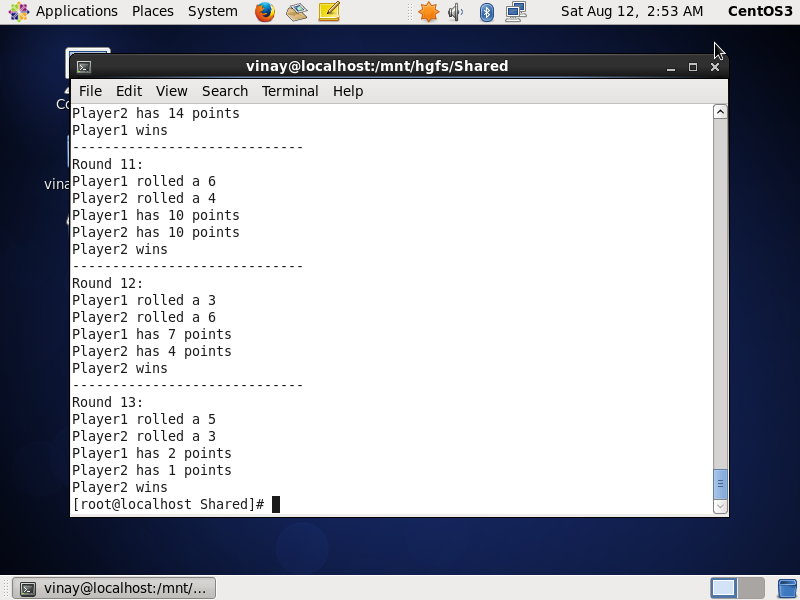# Question & Answer: IN JAVA: This game is meant for two or more players., Each player starts out with 50 points, as each player takes a turn rolling the dic…..

IN JAVA: This game is meant for two or more players., Each player starts out with 50 points, as each player takes a turn rolling the dice; the amount generated by the dice is subtracted from the player’s points. The first player with exactly one point remaining wins. If a player’s remaining points minus the amount generated by the dice results in a value less than one, then the amount should be added to the player’s points. (As a alternative, the game can be played with a set number of turns. In this case the player with the amount of points closest to one, when all rounds have been played, wins.) Write a program that simulates the game being played by two players. Use the Die class that was presented in Chapter 6 to simulate the dice. Write a Player class to simulate the player. Enter the player’s names and display the die rolls and the totals after each round.

THIS IS THE DIE CLASS: please complete entire program and upload screenshot of output along with the answer.

Don't use plagiarized sources. Get Your Custom Essay on
Question & Answer: IN JAVA: This game is meant for two or more players., Each player starts out with 50 points, as each player takes a turn rolling the dic…..
GET AN ESSAY WRITTEN FOR YOU FROM AS LOW AS \$13/PAGE

import java.util.Random;

/**
The Die class simulates a six-sided die.
*/

public class Die
{
private int sides; // Number of sides
private int value; // The die’s value

/**
The constructor performs an initial
roll of the die.
@param numSides The number of sides for this die.
*/

public Die(int numSides)
{
sides = numSides;
roll();
}

/**
The roll method simlates the rolling of
the die.
*/

public void roll()
{
// Create a Random object.
Random rand = new Random();

// Get a random value for the die.
value = rand.nextInt(sides) + 1;
}

/**
getSides method
@return The number of sides for this die.
*/

public int getSides()
{
return sides;
}

/**
getValue method
@return The value of the die.
*/

public int getValue()
{
return value;
}
}

OUTPUT SHOULD BE SOMETHING LIKE:

———————————

Round 1:

player1 rolled a 6

player2 rolled a 4

player1 has 44 points

player2 has 46 points

player1 wins.

———————————-import java.io.*;
import java.util.*;

class Die
{
private int sides;
private int value;

public Die(int numSides)
{
sides = numSides;
roll();
}

public void roll()
{
// Create a Random object.
Random rand = new Random();

// Get a random value for the die.
value = rand.nextInt(sides) + 1;
}

public int getSides()
{
return sides;
}

public int getValue()
{
return value;
}
}

class Player{

private String name;
private int score;
private Die die;
public Player(String a){
name = a;
score = 50;
die = new Die(6);
}
public int roll(){
die.roll();
return (die.getValue());
}
public void modifyScore(int a){
if ((score – a) < 1)
score = score + a;
else
score = score -a;
}
public int getScore(){
return score;
}
public String getName(){
return name;
}

}

public class DemoGame {
public static void main(String[] args){
int s1,s2;
int diff1, diff2;
String name1 , name2;
Scanner sc = new Scanner(System.in);
System.out.println(“Enter Player 1 Name:”);
name1 = sc.next();
System.out.println(“Enter Player 2 Name:”);
name2 = sc.next();

Player p1 = new Player(name1);
Player p2 = new Player(name2);
int count = 1;
while (p1.getScore() != 1 && p2.getScore() != 1){
System.out.println(“—————————–“);
System.out.println(“Round ” + count + “:”);
s1 = p1.roll();
p1.modifyScore(s1);
System.out.println(p1.getName() + ” rolled a ” + s1);
diff1 = p1.getScore() – 1;
s2 = p2.roll();
p2.modifyScore(s2);
System.out.println(p2.getName() + ” rolled a ” + s2);
diff2 = p2.getScore() – 1;
System.out.println(p1.getName() + ” has ” + p1.getScore() + ” points”);
System.out.println(p2.getName() + ” has ” + p2.getScore() + ” points”);
if (diff1 == diff2){
System.out.println(“It is a tie”);
}
else {
if (diff1 < diff2){
System.out.println(p1.getName() +” wins”);
}
else {
System.out.println(p2.getName() + ” wins”);
}
}
count++;
}

}
}